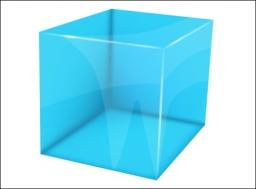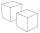# Cube 5

The surface of the cube is 15.36 dm2. How will change the surface area of this cube if the length of the edges is reduced by 2 cm?

S =  11.76 dm2
S1 - S2 =  3.6 dm2

### Step-by-step explanation:Did you find an error or inaccuracy? Feel free to write us. Thank you!Tips to related online calculators
Do you want to convert length units?

## Related math problems and questions:

• Cube edgeDetermine the edges of the cube when the surface is equal to 37.5 cm square.
• Cube edgesThe sum of the lengths of the cube edges is 42 cm. Calculate the surface of the cube.
• CubeThe sum of all cube edges is 30cm. Find the surface area of the cube.
• The cubeThe cube has a surface area of 216 dm2. Calculate: a) the content of one wall, b) edge length, c) cube volume.
• Cube 5The content area of one cube wall is 32 square centimeters. Determine the length of its edges, its surface and volume.
• Cube wallThe surface of the first cube wall is 64 m2. The second cube area is 40% of the surface of the first cube. Determine the length of the edge of the second cube (x).
• Sum of the edgesThe sum of the lengths of all edges of the cube is 72 cm. How many cm2 of colored paper are we going to use for sticking?
• Cube V2SThe volume of the cube is 27 dm cubic. Calculate the surface of the cube.
• Cube 1-2-3Calculate the volume and surface area of the cube ABCDEFGH if: a) /AB/ = 4 cm b) perimeter of wall ABCD is 22 cm c) the sum of the lengths of all edges of the cube is 30 cm.
• The cubeThe cube has a surface of 600 cm2. What is its volume?
• TerezaThe cube has an area of base 256 mm2. Calculate the edge length, volume, and area of its surface.
• CubesSurfaces of cubes, one of which has an edge of 48 cm shorter than the other, differ by 36288 dm2. Determine the length of the edges of this cubes.
• Cube wallsFind the volume and surface area of the cube if the area of one wall is 40cm2.
• Two rectangular boxesTwo rectangular boxes with dimensions of 5 cm, 8 cm, 10 cm, and 5 cm, 12 cm, 1 dm are to be replaced by a single cube box of the same cubic volume. Calculate its surface.
• A butterA butter cube with an edge 6.5 cm long is packed in a package with dimensions a = 28 cm, b = 15 cm. Calculate how many cm2 the package is larger than the surface of the cube.
• Cube 8The surface of the cube is 0.54 m2. Calculate the length of the cube edge.
• Cuboid surfaceDetermine surface area of cuboid if its volume is 52.8 cm cubic and length of the two edges are 2 cm and 6 cm.